## Signature (Recurrence Relation)

Let a sequence be defined by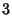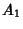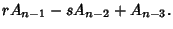Also define the associated Polynomialand let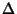be its discriminant. The Perrin Sequence is a special case corresponding to. The signature modof an Integerwith respect to the sequence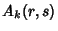is then defined as the 6-tuple (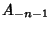,,,,,) (mod).
1. An Integerhas an S-signature if its signature (mod) is (,,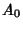,,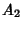).

2. An Integerhas a Q-signature if its signature (mod) is Congruent to () where, for some Integerwith,,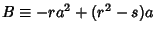, and.

3. An Integerhas an I-signature if its signature (mod) is Congruent to (), whereand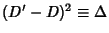.

Adams, W. and Shanks, D. Strong Primality Tests that Are Not Sufficient.'' Math. Comput. 39, 255-300, 1982.
Grantham, J. Frobenius Pseudoprimes.'' http://www.clark.net/pub/grantham/pseudo/pseudo1.ps.